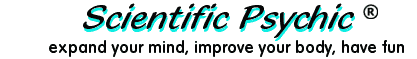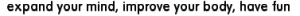Index# Three GenerationsThe grandson is about as many days old as the son is in weeks. The grandson is approximately as many months old as the father is in years. The ages of the grandson, the son, and the father add up to 120 years. What are their ages in years?

Let G, S, and F represent the ages in years of the grandson, the son, and the father, respectively. Since a year has 365 days, or 52 weeks, or 12 months, the problem can be represented by three equations with three unknowns:

365 G = 52 S (The grandson is about as many days old as the son is in weeks)
12 G = F (The grandson is approximately as many months old as the father is in years)
G + S + F = 120 (The ages of the grandson, the son, and the father add up to 120 years)

Because the grandson's age is G = F/12, the son's age can be represented in terms of F by substituting:

S = (365/52)×G
S = (365/52)×(F/12)

The third equation can now be represented with only the father's age as an unknown:

F/12 + (365/52)×(F/12) + F = 120

Multiplying by 12, we get:

F + (365/52)×F + 12 F = 120×12
20 F = 1440
F = 72
G = F/12 = 6
S = (365/52)×6 = 42

The father is 72, the son is 42, and the grandson is 6 years old.

You may have noticed that the statement of the problem is somewhat ambiguous: "The grandson is approximately as many months old as the father is in years" indicates that there may be some variance. A year really has 365 1/4 days instead of 365, and 52 weeks times 7 days gives only 364 days in a year. These inconsistencies produce fractional results that need to be rounded.

Doing the division in the following equation:
F + (365/52)×F + 12 F = 120×12

produces 20.019 F = 1440, which is rounded to 20 F = 1440